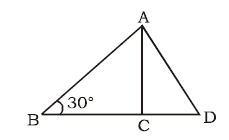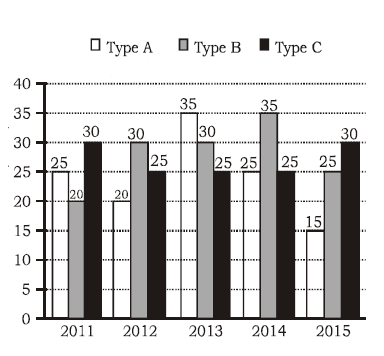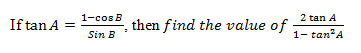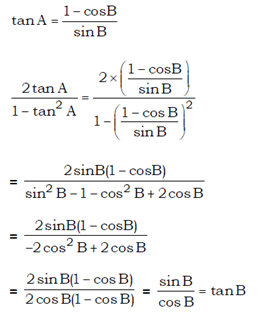# SSC CGL 2018 Practice Test Papers | Quantitative Aptitude (Day-34)

Dear Aspirants, Here we have given the Important SSC CGL Exam 2018 Practice Test Papers. Candidates those who are preparing for SSC CGL 2018 can practice these questions to get more confidence to Crack SSC CGL 2018 Examination.

[WpProQuiz 3260]

Click “Start Quiz” to attend these Questions and view Explanation

1) A water reservoir in a city is normally filled in 8 hours but takes 2 hours longer to fill because of a leak in its bottom. If the reservoir is full, in how many hours will the leak empty it?

a) 34 hours

b) 36 hours

c) 38 hours

d) 40 hours

2) In the figure given below, B = 38°, AC = BC and AD = CD. What is the value of D?a) 26°

b) 28°

c) 24°

d) 30°

(3-6) Study the following graph carefully and answer the question based on it.3) What was the percentage drop in the number of C-type tyres manufactured from 2012 to 2013?

a) 25%

b) 10%

c) 0%

d) None of these

4) What was the difference between the number of B-type tyres manufactured in 2013 and 2014?

a) 100000

b) 2000000

c) 500000

d) 1500000

5) The total number of all the three types of tyres manufactured was the least in which of the following years?

a) 2014

b) 2015

c) 2011

d) 2013

6) In which of the following years was the percentage production of B-types tyres to C-type tyres to maximum?

a) 2013

b) 2011

c) 2015

d) 2014

7)a) tan B/2

b) 2 tan B

c) tan B

d) 4 tan B

8) If p + q = 2, then find the value of p3 + q3 + 6 pq.

a) 8

b) 11

c) 2

d) 6

9) The sum of three numbers is 168. The ratio of second and the third number is 7: 9 and the 1st and the third are as 8: 27, what is second number?

a) 29

b) 16

c) 14

d) 23

10) Simplify: 8456 – 3458 – 2314 + 333

a) 2017

b) 4234

c) 3017

d) None of these

A=1/8

A+B=1/10

B = 1/10 – 1/8 = 1/ 40

The Leak empties the tank in 40 hours.

In ∠ABC

AC = BC

∠BAC = ∠ABC = 38°

∠ACB = 180° – 2 × 38° = 104°

∠ACD = 180° – ∠BCA = 180° – 140°= 76°

In ∠ACD

∠ACD = ∠DAC = 76°

∠D = 180° – 2 × 76° = 28°

Required percentage = (25-25)/25× 100

= 0%

Required difference = (35 – 30) × 100000 = 500000

Number of tyres manufactured in 2011= 75

Number of tyres manufactured in 2013= 90

Number of tyres manufactured in 2015= 60

Number of tyres manufactured in 2014= 85

Production of B is greater than C in three years 2012, 2013 and 2014

Percentage difference in 2012 and 2013= (30-25)/25×100=20%

Percentage difference in 2014= (35-25)/25×100=40%p + q = 2

(p + q)3 = (2)3

p3 + q3 + 3pq (p + q) = 8

p3 + q3 + 3 pq(2) = 8

p3 + q3 + 6 pq = 8

Let three numbers be x, y and z respectively

y:z = 7:9

x:z = 8:27

x:y:z = 8:21:27

8+21+27 = 56

Y = 168/56×21 = 63